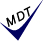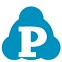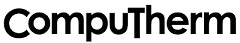## 熱力学データベース　PanNickel```
ソフトウェア Pandat と用いることにより、
ニッケル基合金の平衡状態図を計算できます。　(2007: Ir, Pt, Ru 元素追加）, (2013: Cu, Mn 元素追加)
(2021: P, S, V, Y 元素追加) , (2022: O     元素追加)

ニッケル基合金用（２７元素）     (2022)
Ni, Al, B, C, Co, Cr, Cu, Fe, Hf, Ir, Mn, Mo, N, Nb, O, P, Pt, Re, Ru, S, Si, Ta, Ti, V, W, Y, Zr

PanNickel
Developed for commercial Ni-rich alloys.

Contains more than 342 phases.

Recommended composition range (in wt%):
Al: less than 22
B : less than 0.5
C : less than 0.5
Co: less than 22
Cr: less than 22
Cu: less than 0.5
Fe: less than 22
Hf: less than 5
Ir: less than 12
Mn: less than 0.5
Mo: less than 12
N : less than 0.5
Nb: less than 5
Ni: balance (should be greater than 50)
O : less than 0.5
P : less than 0.01
Pt: less than 40
Re: less than 12
Ru: less than 12
S : less than 0.01
Si: less than 0.5
Ta: less than 12
Ti: less than 5
V : less than 12
W : less than 12
Y : less than 0.5
Zr: less than 0.5

計算結果例

開発元：米国```